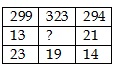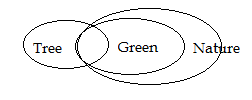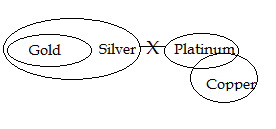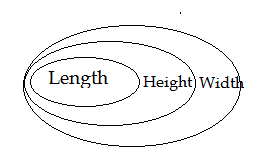# SSC CGL 2018 Practice Test Papers | Reasoning Ability (Day-61)

Dear Aspirants, Here we have given the Important SSC CGL Exam 2018 Practice Test Papers. Candidates those who are preparing for SSC CGL 2018 can practice these questions to get more confidence to Crack SSC CGL 2018 Examination.

[WpProQuiz 3777]

Click “Start Quiz” to attend these Questions and view Explanation

Direction (1 – 3): In the question two statements are given, followed by two conclusions, I and II. You have to consider the statement to be true even if it seems to be at variance from commonly known facts. You have to decide which of the conclusions, if any, follows from the given statement.

1) Statement

Some trees are Green

All greens are Nature

Conclusion:

1. Some nature is Tree
2. No tree is Nature

a) Only I follow

b) Only II follow

c) Both conclusions is follow

d) None of these

2)  Statement:
All Gold is silver

No silver is Platinum

Some platinum is Copper

Conclusion:

1. Some Platinum is Gold
2. No Gold is Platinum

a) Only I follow

b) Only II follow

c) Both conclusions is follow

d) None of these

3) Statement:

All Lengths are height

All Heights are Width

Conclusion:

1. Some widths are length
2. Some Heights are Lengths

a) Only I follow

b) Only II follow

c) Both conclusions is follow

d) None of these

Direction: (4- 5): There are four kids having some chocolates. A have more chocolates than B but less than C. C has 7 chocolates more than D.  B has second least amounts of chocolates.

4) If C has 20 chocolates, what is the possible count of chocolate of B?

a) 13

b) 16

c) 15

d) 18

5) Who is having second most number of chocolate?

a) B

b) D

c) A

d) B

6) Select the missing number from given responsesa) 18

b) 17

c) 15

d) 21

7) In the following question, select the odd word from the given alternatives.

a) Almonds

b) Potato

c) Brinjal

d) Tomato

8) In the following question, select the related number from the given

5 : 36 : : 8 : ?

a) 169

b) 144

c) 64

d) 81

9) In the following question, select the odd word pair from the given alternatives.

a) Time: Seconds

b) Speed: Km per Hour

c) Distance: Meter

d) Time : MillimetreIf C – 20

A

B

D – 14

Then 20 > B > 14

C > A > B > D

299 ÷ 13 = 23

323 ÷ 17 = 19

294 ÷ 21 = 14

Almond is a try fruits. All others are vegetables

5 + 1 = 6 ^2 =36

8 + 1 = 9 ^ 2 = 81